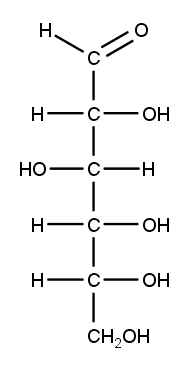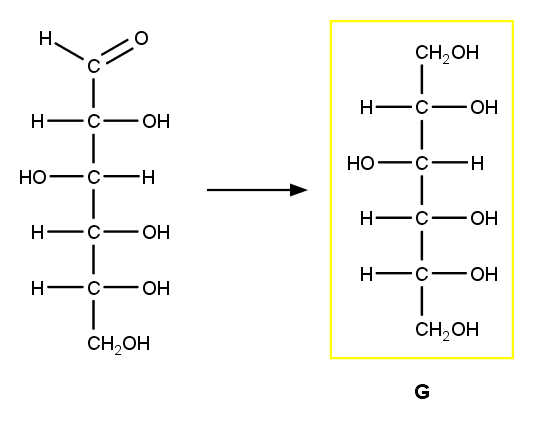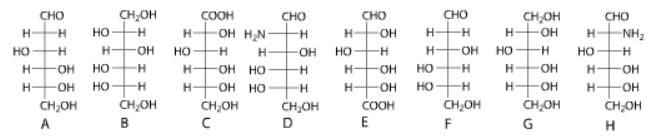# Problem: Identify the glucose derivatives below by selecting the correct structure for each.sorbitol                      a) Ab) Bc) Cd) De) Ef) Fg) Gh) HD-2-deoxyglucose a) Ab) Bc) Cd) De) Ef) Fg) Gh) HD-glucosaminea) Ab) Bc) Cd) De) Ef) Fg) Gh) HD-glucoronic acida) Ab) Bc) Cd) De) Ef) Fg) Gh) H

###### FREE Expert Solution

Fischer Projection of D-Glucose:Sorbitol: aldehyde group of glucose is reduced to an alcohol89% (51 ratings)###### Problem Details

Identify the glucose derivatives below by selecting the correct structure for each.sorbitol

a) A

b) B

c) C

d) D

e) E

f) F

g) G

h) H

D-2-deoxyglucose

a) A

b) B

c) C

d) D

e) E

f) F

g) G

h) H

D-glucosamine

a) A

b) B

c) C

d) D

e) E

f) F

g) G

h) H

D-glucoronic acid

a) A

b) B

c) C

d) D

e) E

f) F

g) G

h) H